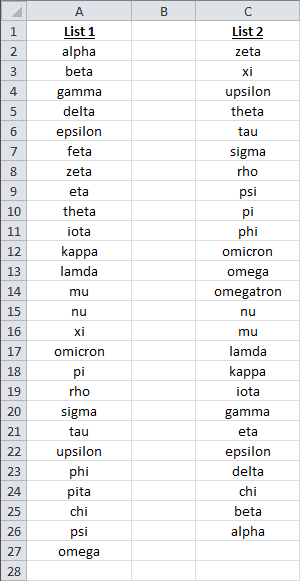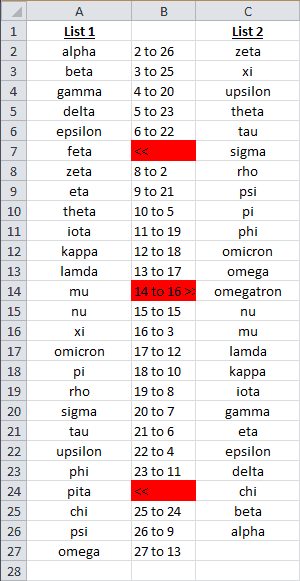Introduction | Example | Tutorial | Applications

## Introduction - Compare Two Excel Columns

Find differences between two excel columns with this VBA tutorial. The VBA module loops through columns of unknown length and unknown order. It compares the longer list to the shorter list, highlights differences and outputs a “key” that points to the matching cells.

## Example - Compare Two Excel Columns

### Find differences between two Excel columns

``````Option Explicit
Sub CompareColumns()
'---------------------------------------------------------------------------------------------------
'---Script: CompareColumns--------------------------------------------------------------------------
'---Created by: Ryan Wells -------------------------------------------------------------------------
'---Date: 03/2015-----------------------------------------------------------------------------------
'---Description: This module loops through two columns in Excel and identifies items without -------
'----------------a match. It also returns a key that points you to the matching partner. -----------
'---------------------------------------------------------------------------------------------------
Dim strCol1 As String 'First Column Location
Dim strCol2 As String 'Second Column Location
Dim strColResults As String 'Output Column
Dim iListStart As Integer 'Row where List Begins
Dim strTemp As String
Dim i As Integer, j As Integer
Dim iLastRow1 As Integer, iLastRow2 As Integer

'---Edit these variables---'
strCol1 = "A"
strCol2 = "C"
strColResults = "B"
iListStart = 2
'--------------------------'

iLastRow1 = ActiveSheet.Range(strCol1 & "50000").End(xlUp).Row
iLastRow2 = ActiveSheet.Range(strCol2 & "50000").End(xlUp).Row
'error check
If iListStart > WorksheetFunction.Min(iLastRow1, iLastRow2) Then
Exit Sub
End If

Range(strColResults & iListStart & ":" & strColResults & _
WorksheetFunction.Max(iLastRow1, iLastRow2)).Clear

strTemp = "<<"
If iLastRow2 > iLastRow1 Then 'switch the order
strTemp = strCol1
strCol1 = strCol2
strCol2 = strTemp
strTemp = ">>"
End If

'Identify unmatched items in long column
For i = iListStart To WorksheetFunction.Max(iLastRow1, iLastRow2)
For j = iListStart To WorksheetFunction.Min(iLastRow1, iLastRow2)
If UCase(Range(strCol2 & j)) = UCase(Range(strCol1 & i)) Then
Range(strColResults & i) = i & " to " & j
Exit For ' Stops at first match
ElseIf j = WorksheetFunction.Min(iLastRow1, iLastRow2) Then
Range(strColResults & i) = strTemp
Range(strColResults & i).Interior.Color = 255
End If
Next j
Next i

'Identify unmatched items in short column
If strTemp = "<<" Then
strTemp = " >>"
Else
strTemp = " <<"
End If
For i = iListStart To WorksheetFunction.Min(iLastRow1, iLastRow2)
For j = iListStart To WorksheetFunction.Max(iLastRow1, iLastRow2)
If UCase(Range(strCol1 & j)) = UCase(Range(strCol2 & i)) Then
Exit For
ElseIf j = WorksheetFunction.Max(iLastRow1, iLastRow2) Then
Range(strColResults & i) = Range(strColResults & i) & strTemp
Range(strColResults & i).Interior.Color = 255
End If
Next j
Next i

End Sub``````

Make powerful macros with our free VBA Developer Kit

This is actually pretty neat. If you have trouble understanding or remembering it, our free VBA Developer Kit can help. It’s loaded with VBA shortcuts to help you make your own macros like this one - we’ll send a copy, along with our Big Book of Excel VBA Macros, to your email address below.

I'll take a free VBA Developer Kit

## Tutorial - Compare Two Excel Columns

Let’s set the stage. You have two columns of data. They could be anything - file checksums, directory structures, invoices, email addresses, you name it. You want to compare the columns but they are different lengths. Not only that, the ranges are sorted differently. Your goal is to identify which values in “Column 1” are not on “Column 2” and vice versa.

The CompareColumns subroutine solves your problem.BeforeAfter

You mean Feta, Pita and Omegatron are not in the Greek alphabet?

In addition to highlighting the differences between the columns, the module points to the list with the extra item (« or »).

If a match is found between the two columns, CompareColumns returns a “key” that directs you to the matching item. For instance, cell “B19” tells you row 19 in List 1 matches row 8 in List 2. This feature is useful if you need to perform additional post-processing on your lists. You can simply split the key integers and use them later.

It’s important to know CompareColumns always compares the long column to the short column. Therefore, the first value in the key will correspond to the row in the longer list.

The macro performs a case-insensitive search. If you desire case-sensitivity, remove the `UCase` function in the `If` statements.

There are only 4 variables you’ll need to edit to get this code up and running:

1. strCol1
• a string representing the column where your first list is stored.
2. strCol2
• a string representing the column where your second list is stored.
3. strColResults
• a string representing the column where you want your output key to be displayed.
4. iListStart
• the integer row where your list begins.

Check out this End(xlUp).Row tutorial to learn how to customize the LastRow integers in the subroutine.

## Application Ideas - Compare Two Excel Columns

I regularly use a variation of this comparison tool to validate checksums. I modify my `If` statements to use the InStr command.

I’ll start with two lists of cksum outputs. Each output is pasted into Excel. I want the program to compare the list with unique file names to the list with directories and file names. If the filename is found in the directory path string, the program will offset 2 columns to confirm the file checksums match. This is one example of why the “key” is useful.

That’s all for this tutorial. When you’re ready to take your VBA to the next level, subscribe using the form below.